# Extracting square roots mentally at an extremely fast speedFinding the square root of a number is the inverse operation of squaring that number. Remember, the square of a number is that number times itself.

Square of 5 = 52 = 5 × 5 = 25. Therefore, the square root of 25 is 5. The perfect squares are the squares of the whole numbers such as 1, 4, 9, 16, 25, 36, 49, 64, 81, 100 so on and so forth.

Before learning the procedure, it is wise that the performer memorizes the squares of the numbers 1-10 which is very elementary.

To extract the square root of any perfect square, follow the steps presented below:

Step-1:  Look at the magnitude of the “hundreds number” (the numbers preceding the last two digits) and find the largest square that is equal to or less than the number. This is the 1stpart of the answer.

Step-2:  Now, look at the last (unit’s) digit of the number. If the number ends in a:

0 -> then the ending digit of the answer is a 0
1 -> then the ending digit of the answer is 1 or 9.
4 -> then the ending digit of the answer is 2 or 8.
5 -> then the ending digit of the answer is a 5.
6 -> then the ending digit of the answer is 4 or 6.
9 -> then the ending digit of the answer is 3 or 7.

To determine the right answer from 2 possible answers (other than 0 and 5), mentally multiply the findings in step-1 with its next higher number. If the left extremities (the numbers preceding the last two digits) are greater than the product, the right digit would be the greater option (9,8,7,6) and if left extremities are less than the product, the right digit would be the smaller option (1,2,3,4).

Let us illustrate the trick with some examples:

Extracting square root of 784 (√784)
1. Look at the magnitude of the “hundreds number” (the numbers preceding the last two digits) which is 7. Now, 22=4 and 32=9. So, the highest square in 7 is 2 which is the 1stpart of the answer.
2. Now, look at the last digit of the number which is 4. We know if the number ends in a 4 then the ending digit of the answer would be 2 or 8.
Now, 2 (findings in step-1) times its next higher number which is 3 is (2×3=) 6. The left extremity which is 7 is greater than 6. Therefore, the right digit of the answer must be the greater option which is 8.

So, our final answer is 28.

Let’s go for another example: √3969 (square root of 3969)
1. The magnitude of the “hundreds number” is 39. Now, 62=36 and 72=49. So, the highest square in 39 is 6.
2. Looking at the last digit of the number which is 9; we know if the number ends in a 9 then the last digit of the answer would be 3 or 7.
Now, 6 (findings in step-1) times its next higher number 7 is (6×7=) 42. And 39 (the left extremities) is less than 42. Therefore, the right digit of the answer must be the smaller option i.e. 3.

So, our final answer is 63.

So, Square root of 5476 (√5476) = ?
1. The numbers preceding the last two digits is 54; the highest square in it is 7.
2. The last digit of the number is 6 so; the ending digit of the answer would be 4 or 6.
Now, 7 times its next higher number (8) is 56. Since 54 is less than 56, the right digit of the answer must be the smaller option i.e. 4.

So, our final answer is 74.

Square root of 13689 (√13689) = ?
1. Focusing 136; the highest square in it is 11 (since, 112 = 121 and 122 = 144).
2. The last digit of the number is 9 so; the ending digit of the answer would be 3 or 7.
11 times its next higher number (12) is 132 and 136 is greater than 132, so the right digit of the answer would be 7.

So, the final answer is 117.

Square root of 15376 (√15376) = ?
1. The highest square in 153 is 12 (122 = 144 and 132 = 169).
2. The last digit of the number 6 makes the ending digit of the answer a possibility of 4 or 6.
12 times its next higher number (13) is 156. Since 153 is less than 156, the right digit of the answer must be 4 giving the final answer 124.

1.Great post ...Keep it up Sir !!

2.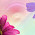will this applicable to any numbers like sq.root of 1471369 ? please explain ....

1.1471369 does work, 121 is the largest root of 14713, the possible roots of 9 is 3 and 7, 121*122 is 14762, which is bigger than 14713, so you use the smaller root of 9, resulting in 1213.

3.Nice to see that blog commenting done right still has many benefits. Thanks for sharing your insights.

Math Problems Online

4.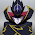How did you make the heading?
Burning maths

1.animated headings or fonts!

5.The shortcut trick really work. Is there any proof why the trick works? please share thanks

6.You just ensured I will do well on the Math eogs

7.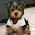Root of 161 and 289 by this method ?

8.How does it works on 8 digit perfect square?

9.how 2 find the approx cube root of 636000

10.What if the number is not a perfect square?

11.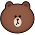It doesn't work when i try to find the square root of 324!!

1.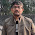IT DOES WORK for 324 , it works for perfect squares

2.yes. it works . you get 12 as an answer so u got an idea that its somewhere near 12.

3.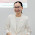How to know if it is a perfect square?

12.Nice tricks. Helped a lot. Thank you😀

13.Please tell me how to know if it's a perfect square.

14.Thank you for the short cut. This will have a great help for me...

15.Sonica,
There's a tutorial on this namely 'How to check if a number is a Perfect Square', Plz check

16.Thank you.it was helpful!

17.Awesome sir keep it up

18.that's perfect answer by you thanks for the great post. its was so helpful to all.

19.awesome trick really works....practically done
keep doing this

20.Awesome Sir! Keep posting such tricks.

21.Awesome Trick ...Really You saved My life

22.Hello thanks for post-so does this mean that numbers ending in 2, 3, 7 & 8 are not perfect squares? Thx

23.## Mental Math for Faster Calculation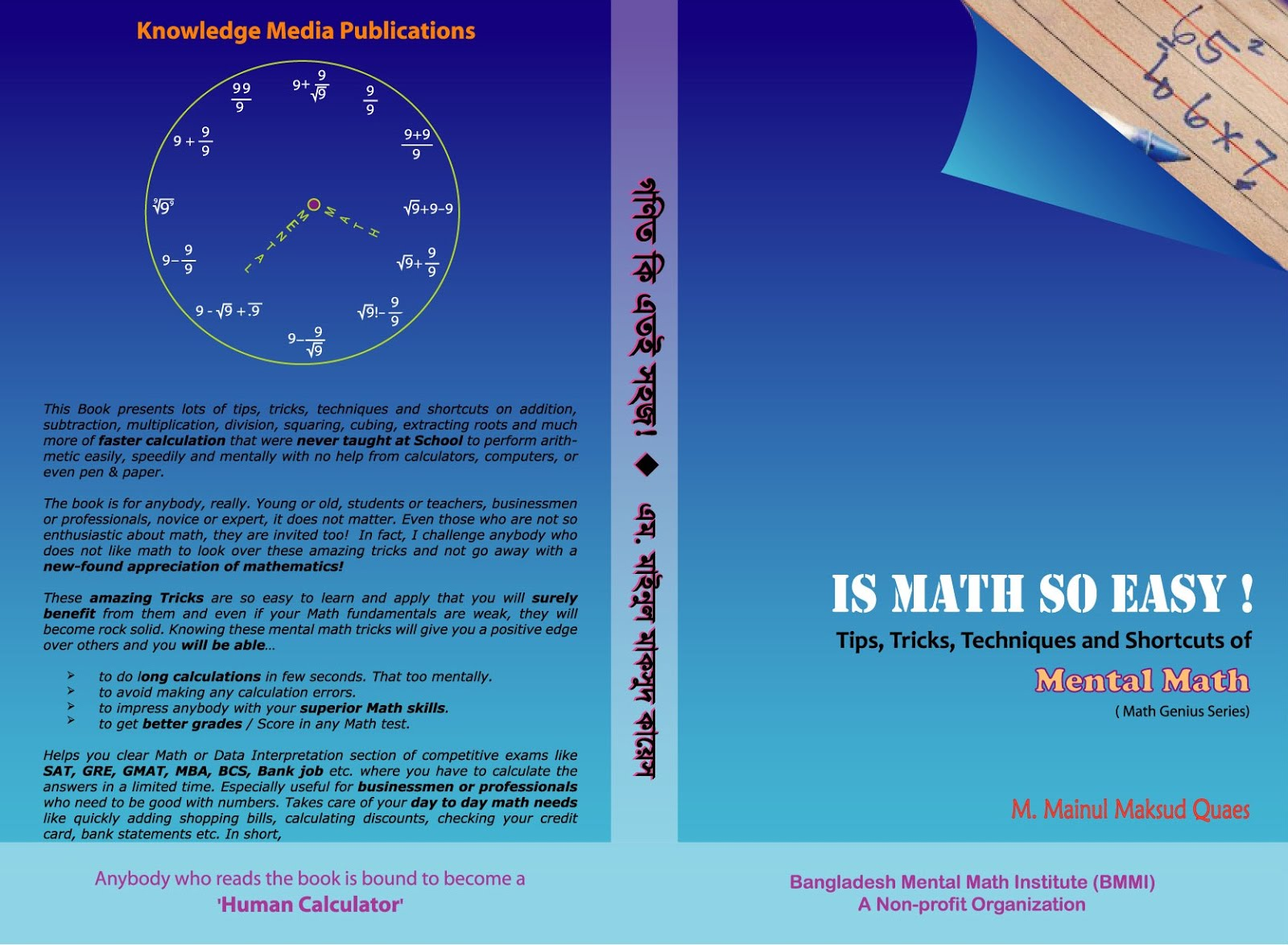© 2013-16 Burning Math